Next: Heat capacity or specific Up: Classical thermodynamics Previous: Introduction

## The equation of state of an ideal gas

Let us start our discussion by considering the simplest possible macroscopic system: i.e., an ideal gas. All of the thermodynamic properties of an ideal gas are summed up in its equation of state, which determines the relationship between its pressure, volume, and temperature. Unfortunately, classical thermodynamics is unable to tell us what this equation of state is from first principles. In fact, classical thermodynamics cannot tell us anything from first principles. We always have to provide some information to begin with before classical thermodynamics can generate any new results. This initial information may come from statistical physics (i.e., from our knowledge of the microscopic structure of the system under consideration), but, more usually, it is entirely empirical in nature (i.e., it is the result of experiments). Of course, the ideal gas law was first discovered empirically by Robert Boyle, but, nowadays, we can justify it from statistical arguments. Recall (from Sect. 3.8), that the number of accessible states of a monotonic ideal gas varies like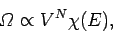(265)

whereis the number of atoms, anddepends only on the energy of the gas (and is independent of the volume). We obtained this result by integrating over the volume of accessible phase-space. Since the energy of an ideal gas is independent of the particle coordinates (because there are no interatomic forces), the integrals over the coordinates just reduced tosimultaneous volume integrals, giving the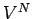factor in the above expression. The integrals over the particle momenta were more complicated, but were clearly completely independent of, giving thefactor in the above expression. Now, we have a statistical rule which tells us that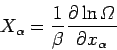(266)

[see Eq. (197)], whereis the mean force conjugate to the external parameter(i.e.,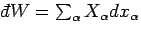), and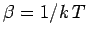. For an ideal gas, the only external parameter is the volume, and its conjugate force is the pressure (since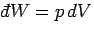). So, we can write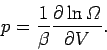(267)

If we simply apply this rule to Eq. (265), we obtain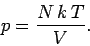(268)

However,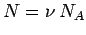, whereis the number of moles, and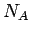is Avagadro's number. Also,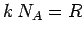, whereis the ideal gas constant. This allows us to write the equation of state in its usual form(269)

The above derivation of the ideal gas equation of state is rather elegant. It is certainly far easier to obtain the equation of state in this manner than to treat the atoms which make up the gas as little billiard balls which continually bounce of the walls of a container. The latter derivation is difficult to perform correctly because it is necessary to average over all possible directions of atomic motion. It is clear, from the above derivation, that the crucial element needed to obtain the ideal gas equation of state is the absence of interatomic forces. This automatically gives rise to a variation of the number of accessible states withandof the form (6.6), which, in turn, implies the ideal gas law. So, the ideal gas law should also apply to polyatomic gases with no interatomic forces. Polyatomic gases are more complicated that monatomic gases because the molecules can rotate and vibrate, giving rise to extra degrees of freedom, in addition to the translational degrees of freedom of a monatomic gas. In other words,, in Eq. (265), becomes a lot more complicated in polyatomic gases. However, as long as there are no interatomic forces, the volume dependence of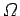is still, and the ideal gas law should still hold true. In fact, we shall discover that the extra degrees of freedom of polyatomic gases manifest themselves by increasing the specific heat capacity.

There is one other conclusion we can draw from Eq. (265). The statistical definition of temperature is [Eq. (187)](270)

It follows that(271)

We can see that since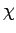is a function of the energy, but not the volume, then the temperature must be a function of the energy, but not the volume. We can turn this around and write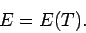(272)

In other words, the internal energy of an ideal gas depends only on the temperature of the gas, and is independent of the volume. This is pretty obvious, since if there are no interatomic forces then increasing the volume, which effectively increases the mean separation between molecules, is not going to affect the molecular energies in any way. Hence, the energy of the whole gas is unaffected.

The volume independence of the internal energy can also be obtained directly from the ideal gas equation of state. The internal energy of a gas can be considered as a general function of the temperature and volume, so(273)

It follows from mathematics that(274)

where the subscriptreminds us that the first partial derivative is taken at constant volume, and the subscript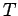reminds us that the second partial derivative is taken at constant temperature. Thermodynamics tells us that for a quasi-static change of parameters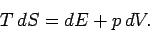(275)

The ideal gas law can be used to express the pressure in term of the volume and the temperature in the above expression. Thus,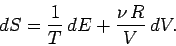(276)

Using Eq. (6.15), this becomes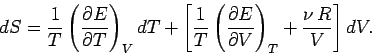(277)

However,is the exact differential of a well-defined state function,. This means that we can consider the entropy to be a function of temperature and volume. Thus,, and mathematics immediately tells us that(278)

The above expression is true for all small values ofand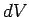, so a comparison with Eq. (277) gives(279)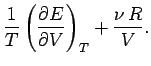(280)

One well-known property of partial differentials is the equality of second derivatives, irrespective of the order of differentiation, so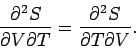(281)

This implies that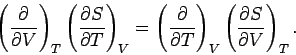(282)

The above expression can be combined with Eqs. (279) and (280) to give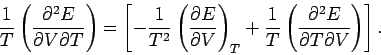(283)

Since second derivatives are equivalent, irrespective of the order of differentiation, the above relation reduces to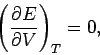(284)

which implies that the internal energy is independent of the volume for any gas obeying the ideal equation of state. This result was confirmed experimentally by James Joule in the middle of the nineteenth century.Next: Heat capacity or specific Up: Classical thermodynamics Previous: Introduction
Richard Fitzpatrick 2006-02-02